# Introduction to Writing Algebraic Terms

Introduction to Writing Algebraic Terms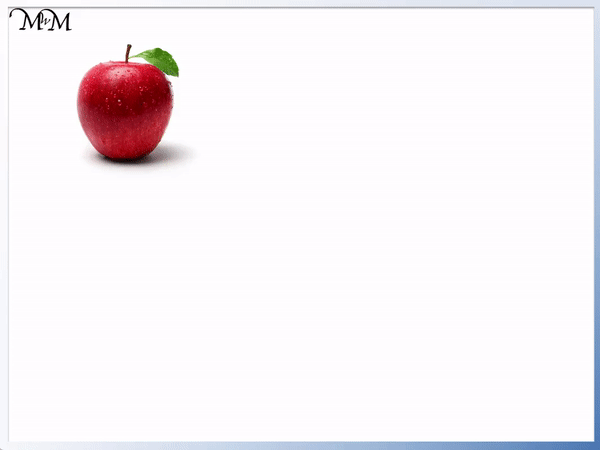• We use letters in algebra when we don’t know the value of something.
• These letters can be called variables.
• We don’t know how much each apple costs, so we say each apple costs ‘a’.
• The total cost of three of these apples is a + a + a, which is 3 lots of a.
• We don’t write the multiplication sign in algebra because we use the letter ‘x’ a lot which looks similar.
• We write a + a + a as 3a, which means that there are 3 a’s.
• We always write the number in front of the letter.

We do not write multiplication signs with algebra.

We write how many of each letter there are with a number in front of the letter.• Here is b + b + b.
• We have 3 b’s in total.
• We can write b + b + b as 3b.
• We don’t write 3 × b because we don’t write multiplication signs in algebra.
• We always write the number in front of the letter, so we write 3b and not b3.# Introduction to Writing Algebra

## What are Algebraic Terms?

Algebraic terms are the different letters or numbers in an expression that are separated by plus or minus signs. A single term can even contain multiple different letters and numbers multiplied together. As long as there is not a plus or minus sign, then it is an algebraic term.

The letters in a term are called variables and the numbers in front of them are called coefficients.

For example, in the expression: c + c + c + c, we have 4 algebraic terms. Each letter ‘c’ is separated by a plus sign, so each letter ‘c’ is a separate term.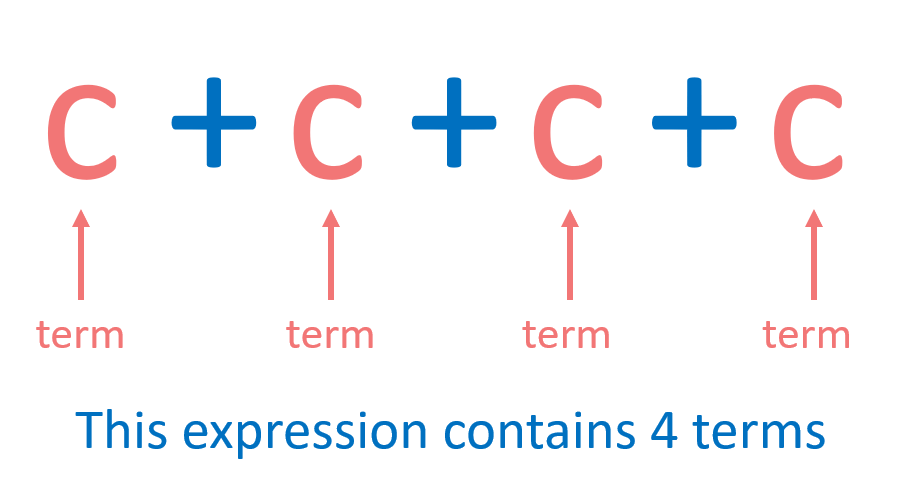In most cases, the algebraic terms will be different letters, such as in the next example below.

It is important to note that even numbers on their own can be algebraic terms. It is not necessary to have an algebraic variable present for it to be classed as a term. In this example of d – 3 + e, we have 3 terms.The first term is ‘d’, the second term is ‘3’ and the third term is ‘e’.

We can see that in this example, the terms are separated by both plus and minus signs.

Remember, if there is not a plus or minus sign separating the letters or numbers, then it is one single term.

For example in the expression: 2a – 4cd there are 2 terms. The first term is ‘2a’ and the second term is ‘4cd’.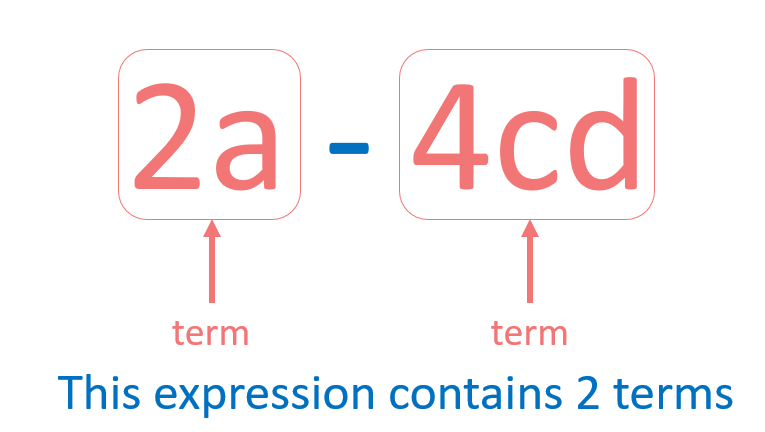## How to Write Algebraic Terms

When writing algebraic terms use the following rules:

• Do not write multiplication signs.
• Write the number in front of the letters in each term.
• If there is only one of a certain algebraic term, do not write the number 1 in front of it. Simply write the letters only.

For example, here is the expression 𝑥 + 𝑥. There are two 𝑥 terms.

𝑥 + 𝑥 is the same as 2 × 𝑥.

The multiplication sign of ‘×’ and the variable ‘𝑥’ look very similar. 𝑥 is a letter that is commonly used in algebra. For this reason, we do not use the multiplication sign in algebra. Removing the multiplication sign also keeps the algebraic expressions shorter and simpler to work with.

Instead of 2 × 𝑥 we write 2𝑥.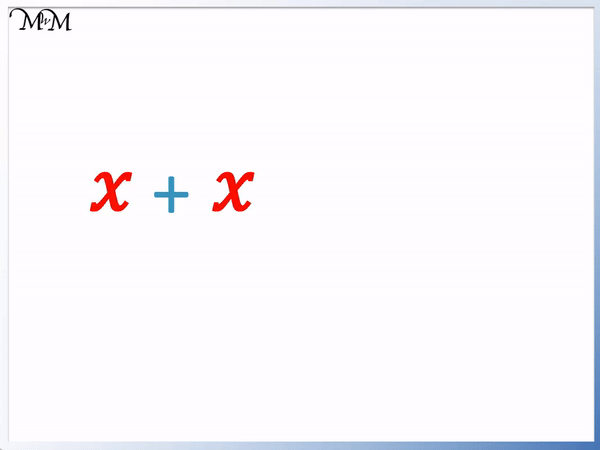2𝑥 means that we have 2 × 𝑥, or 2 x’s.

An important rule used when writing algebraic terms is to avoid using the number 1 in front of an algebraic variable if there is only 1 of them.

For example, here is the variable 𝑥.

Although we only have 1 𝑥, we simply write 𝑥.

We do not write 1𝑥.The reason we do not write 1 in front of algebraic terms is because we don’t need to. It is quicker and easier, especially in longer algebraic expressions to avoid using the number 1. It is easier to write 𝑥 than it is to write 1𝑥.

This is just a simple example and when it comes to more complex expressions and equations, it can be helpful to remove unnecessary information.

In this example, we have y + y + y + y + y.

We have 5 ‘y’ terms, which is 5 lots of y, or 5 × y.

We do not write the multiplication symbol and so, y + y + y + y + y = 5y.5y simply means that we have 5 y terms.

We can see that it is quicker to write 5y than it is to write y + y + y + y + y.

We can do this because all of the terms are y terms. There is no other letter in the expression.

Counting up the same letter terms is known as collecting like terms and is explored in a different lesson Collecting Like Terms.

Here is another example of collecting like terms.

In this expression, we have b + b + b.We have 3 b terms, so we write this as 3b.

Remember that we do not write the multiplication sign and we always write the number in front of the letters.

We write 3b, not b3.

The number that comes in front of the algebraic variables (letters) is called the coefficient.In the example of 5ab, 5ab is one term. ‘5’ is the coefficient and both ‘a’ and ‘b’ are the variables.

## Writing the Letter 𝑥 in Algebra

In this example we have: 𝑥 + 𝑥 + 𝑥 + 𝑥.

We have 4 𝑥’s in total or 4 × 𝑥.

Remember, we do not write the multiplication sign so we simply write 𝑥 + 𝑥 + 𝑥 + 𝑥 as 4𝑥.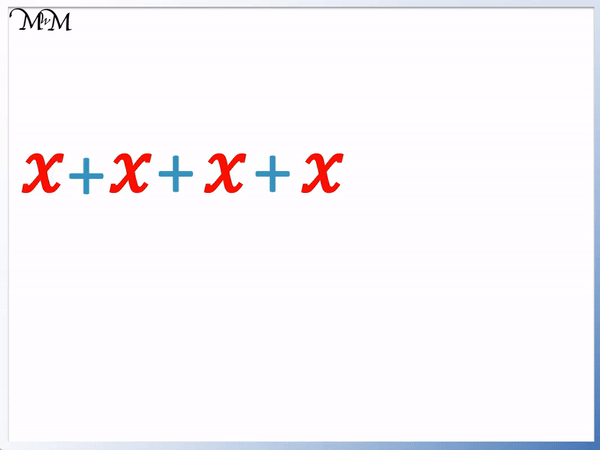𝑥 is a letter commonly used in algebra. You may notice that 𝑥 is written differently to the letter x on your keyboard.

In algebra, 𝑥 is written differently to the usual letter x. The letter x looks similar to the multiplication sign, ×. The symbol of 𝑥 is used instead so that it is clearer that we are not doing a multiplication.

To write the algebra 𝑥, write a letter c and then write a letter c backwards just before it.

The algebra 𝑥 is more curved than the standard letter x.

## What are Algebraic Variables?

Variables are the letters used in algebra that hold the place of numbers. They are used when the value of something is unknown or can vary, hence the name variable.

For example if the price of an apple is $2, then we can work out the price of three apples by multiplying$2 by 3.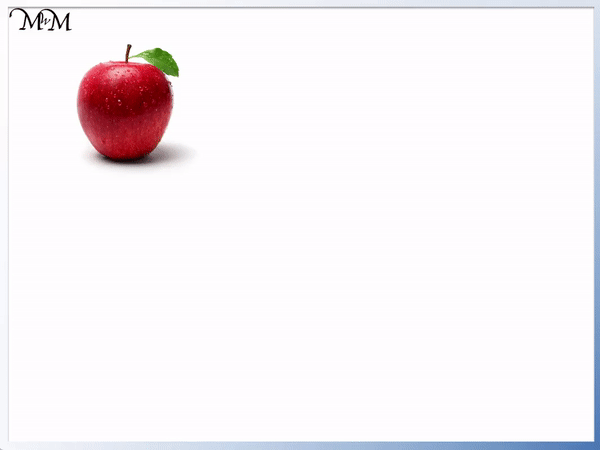$2 × 3 =$6. The total cost would be $6. However, you might pick up the three apples when shopping and not know what price they are. You may only find out the price when someone tells you as you go to pay. Because we do not know the price of each apple, we can use an algebraic variable. We can use the letter ‘a’ to represent the price of an individual apple. Any letter can be used, however I chose ‘a’ because it is apples that we are buying. If we were buying bananas, I could still use ‘a’ but I would probably choose ‘b’ to help remind myself what the letter represents.We have 3 apples, so the total price is a + a + a. We have 3 apples, so the total price is 3 × a. Remember that we do not use multiplication signs in algebra and so we would just write this as 3a. We say that the total price is 3a. When teaching or introducing algebra for the first time, people can be uncomfortable with not having a numerical answer. It helps to explain how the answers in these cases will look like and what they mean. The answer in this case is 3a. If we later find out the price of an apple is$4, then we can use the algebraic term of ‘3a’ to work out the price.

If a = $4, then 3a means 3 ×$4.

If the price of one apple is $4, then the total price of 3 apples would be$12.

The letter ‘a’ is used to hold the value of the apple until we know what it is.Now try our lesson on Negative Numbers on a Number Line where we introduce negative numbers.error: Content is protected !!